Next: 2.3.3.6 Current Source Up: 2.3.3 Devices Previous: 2.3.3.4 Non-linear Capacitor

### 2.3.3.5 Linear Inductor

The constitutive relation for a linear inductor is V = L . dI/dt. For the backward Euler discretization scheme the equations are as follows
 fI = V - R .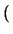I - Io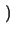= 0 (2.40) R =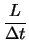(2.41)

and the stamp is given as
 yx, y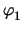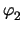I f n1 1 - I n2 -1 I I -1 1 R fI

It is to note that the current I could be eliminated from the stamp given above as demonstrated for the resistor. However, the above formulation has the advantage that for operating point calculations the inductor can easily be replaced by an ideal voltage source with R = 0 and the voltage being the initial condition of the device without a topological change in the circuit.Next: 2.3.3.6 Current Source Up: 2.3.3 Devices Previous: 2.3.3.4 Non-linear Capacitor
Tibor Grasser
1999-05-31